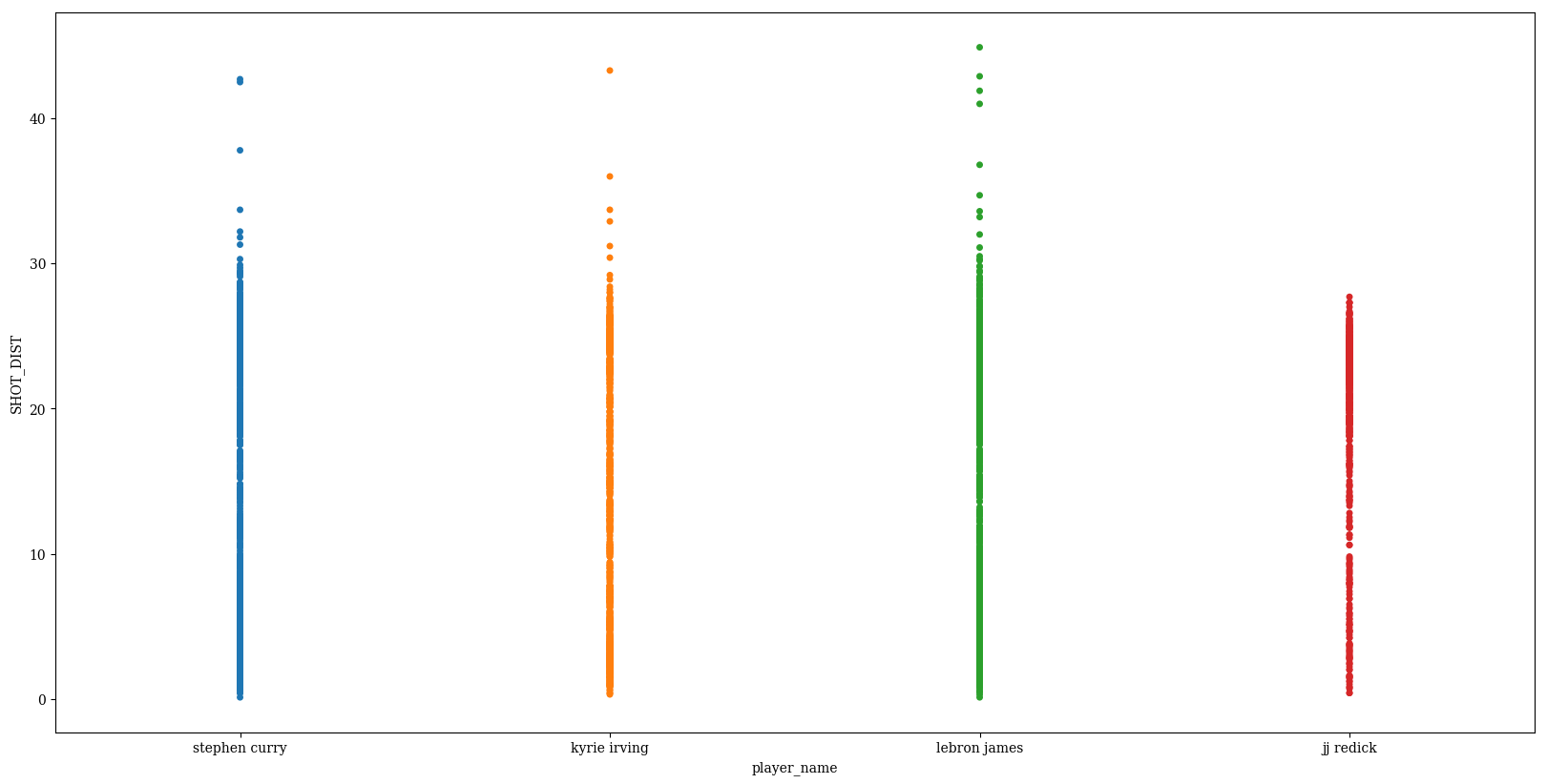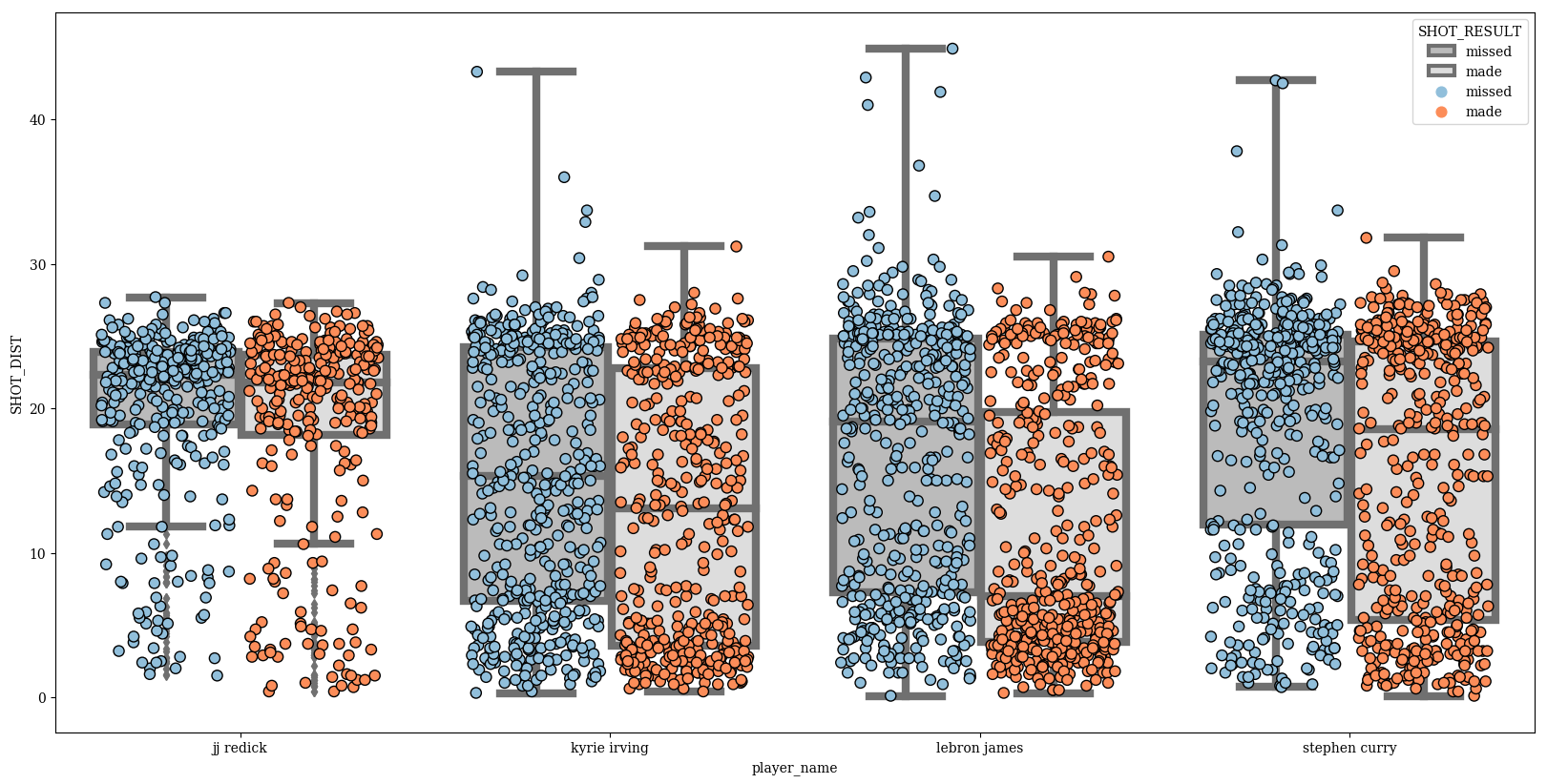# seaborn.stripplot

A strip plot is a scatter plot where one of the variables is categorical. They can be combined with other plots to provide additional information. For example, a boxplot with an overlaid strip plot becomes more similar to a violin plot because some additional information about how the underlying data is distributed becomes visible. Seaborn’s `swarmplot` is virtually identical except that it prevents datapoints from overlapping.

dataset: Kaggle: NBA shot logs

``````%matplotlib inline
import pandas as pd
import matplotlib.pyplot as plt
import seaborn as sns
import numpy as np
plt.rcParams['figure.figsize'] = (20.0, 10.0)
plt.rcParams['font.family'] = "serif"
``````

``````df = pd.read_csv('../stripplot/shot_logs.csv',usecols=['player_name','SHOT_DIST','PTS_TYPE','SHOT_RESULT'])
players_to_use = ['kyrie irving', 'lebron james', 'stephen curry', 'jj redick']
df = df.loc[df.player_name.isin(players_to_use)]
``````
SHOT_DIST PTS_TYPE SHOT_RESULT player_name
14054 8.0 2 missed stephen curry
14055 25.9 3 missed stephen curry
14056 23.8 3 made stephen curry
14057 27.5 3 made stephen curry
14058 29.3 3 missed stephen curry

Basic plot

``````p = sns.stripplot(data=df, x='player_name', y='SHOT_DIST')
``````Change the `color` to represent whether the shot was made or missed

``````p = sns.stripplot(data=df,
x='player_name',
y='SHOT_DIST',
hue='SHOT_RESULT')
``````Change the `order` in which the names are displayed

``````p = sns.stripplot(data=df,
x='player_name',
y='SHOT_DIST',
hue='SHOT_RESULT',
order=sorted(players_to_use))
```````jitter` can be used to randomly provide displacements along the horizontal axis, which is useful when there are large clusters of datapoints

``````p = sns.stripplot(data=df,
x='player_name',
y='SHOT_DIST',
hue='SHOT_RESULT',
order=sorted(players_to_use),
jitter=0.25)
``````We see the default behavior is to stack the different hues on top of each other. This can be avoided with `dodge` (formerly called `split`)

``````p = sns.stripplot(data=df,
x='player_name',
y='SHOT_DIST',
hue='SHOT_RESULT',
order=sorted(players_to_use),
jitter=0.25,
dodge=True)
``````Flipping x and y inputs and setting `orient` to ‘h’ can be used to make a horizontal plot

``````p = sns.stripplot(data=df,
y='player_name',
x='SHOT_DIST',
hue='SHOT_RESULT',
order=sorted(players_to_use),
jitter=0.25,
dodge=False,
orient='h')
``````For coloring, you can either provide a single color to `color`

``````p = sns.stripplot(data=df,
y='player_name',
x='SHOT_DIST',
hue='SHOT_RESULT',
order=sorted(players_to_use),
jitter=0.25,
dodge=True,
orient='h',
color=(.25,.5,.75))
``````…or you can use one of the many variations of the `palette` parameter

``````p = sns.stripplot(data=df,
x='player_name',
y='SHOT_DIST',
hue='SHOT_RESULT',
order=sorted(players_to_use),
jitter=0.25,
dodge=True,
palette=sns.husl_palette(2, l=0.5, s=.95))
``````Adjust the marker `size`

``````p = sns.stripplot(data=df,
x='player_name',
y='SHOT_DIST',
hue='SHOT_RESULT',
order=sorted(players_to_use),
jitter=0.25,
dodge=True,
palette=sns.husl_palette(2, l=0.5, s=.95),
size=8)
``````Adjust the `linewidth` of the edges of the circles

``````p = sns.stripplot(data=df,
x='player_name',
y='SHOT_DIST',
hue='SHOT_RESULT',
order=sorted(players_to_use),
jitter=0.25,
dodge=True,
palette=sns.husl_palette(2, l=0.5, s=.95),
size=8,
linewidth=3)
``````Change the color of these lines with `edgecolor`

``````p = sns.stripplot(data=df,
x='player_name',
y='SHOT_DIST',
hue='SHOT_RESULT',
order=sorted(players_to_use),
jitter=0.25,
dodge=True,
palette=sns.husl_palette(2, l=0.5, s=.95),
size=8,
linewidth=3,
edgecolor='blue')
``````Swarmplots look good when overlaid on top of another categorical plot, like `boxplot`

``````params = dict(data=df,
x='player_name',
y='SHOT_DIST',
hue='SHOT_RESULT',
#jitter=0.25,
order=sorted(players_to_use),
dodge=True)
p = sns.stripplot(size=8,
jitter=0.35,
palette=['#91bfdb','#fc8d59'],
edgecolor='black',
linewidth=1,
**params)
p_box = sns.boxplot(palette=['#BBBBBB','#DDDDDD'],linewidth=6,**params)
``````Finalize

``````plt.rcParams['font.size'] = 30
params = dict(data=df,
x='player_name',
y='SHOT_DIST',
hue='SHOT_RESULT',
#jitter=0.25,
order=sorted(players_to_use),
dodge=True)
p = sns.stripplot(size=8,
jitter=0.35,
palette=['#91bfdb','#fc8d59'],
edgecolor='black',
linewidth=1,
**params)
p_box = sns.boxplot(palette=['#BBBBBB','#DDDDDD'],linewidth=6,**params)
handles,labels = p.get_legend_handles_labels()
#for h in handles:
#    h.set_height(3)
#handles.set_linewidth(33)

plt.legend(handles[2:],
labels[2:],
bbox_to_anchor = (.3,.95),
fontsize = 40,
markerscale = 5,
frameon=False,
labelspacing=0.2)
plt.text(1.85,35, "Strip Plot", fontsize = 95, color='Black', fontstyle='italic')
plt.xlabel('')
plt.ylabel('Shot Distance (ft)')
plt.gca().set_xlim(-0.5,3.5)
xlabs = p.get_xticklabels()
xlabs.set_text('JJ Redick')
for l in xlabs[1:]:
l.set_text(" ".join(i.capitalize() for i in l.get_text().split() ))
p.set_xticklabels(xlabs)
``````
``````[<matplotlib.text.Text at 0x1164fceb8>,
<matplotlib.text.Text at 0x113b96588>,
<matplotlib.text.Text at 0x113abd4e0>,
<matplotlib.text.Text at 0x113abde10>]
````````````p.get_figure().savefig('../../figures/stripplot.png')
``````

A fair bit of information is conveyed with a plot like this. JJ Redick is a shooting guard, and you see most of his shots are from a significant distances, whereas Lebron James has unsurprisingly a lot more attempts at close range. The median for Lebron’s made shots is significantly lower than that for his misses, which is likely a result of him having many points from high percentage close shots/layups. There are a few outlying shots from very high distances, essentially all misses, that most likely are right before a buzzer.

``````
``````